Want to share your content on R-bloggers? click here if you have a blog, or here if you don't.On my previous post Advanced Survey Design and Application to Big Data I mentioned unsupervised learning can be used to generate a stratification variable. In this post I want to elaborate on this point and how they can work together to improve estimates and training data for predictive models.

## SRS and stratified samples

Consider the estimators of the total from an SRS and stratified sample.The variance of these estimators are given byThe variance of the stratification estimator is made up of two components, the within and between strata sum of squares.With some algebra it can be shown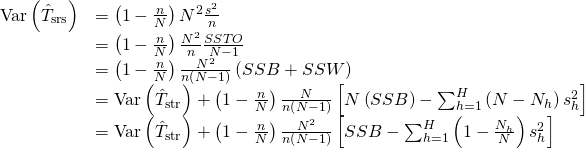This result shows that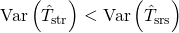while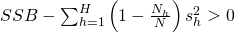0″ title=”Rendered by QuickLaTeX.com” height=”33″ width=”235″ style=”vertical-align: -12px;”/> and asincreases the stratified estimator improves on the SRS estimator.

## Unsupervised Learning

Unsupervised learning attempts to uncover hidden structure in the observed data by sorting the observations into a chosen number of clusters. The simplest algorithm to do this is k-means. The k-means algorithm is as follows:

1. Choose(number of clusters)
2. Chooserandom points and assign as centers3. Compute the distance between each point and each center
4. Assign each observation to the center they are closest to
5. Compute the new centers given the cluster allocation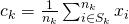where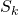contains the points allocated to cluster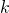6. Compute the between and within sum of squares
7. Repeat 3-6 until the clusters do not change, meet a specified tolerance or max iterations met

The algorithm will minimise the within sum of squares and maximise the between sum of squares.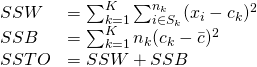As we saw from the formula above the estimator under a stratified sample performs better than an SRS when\sum^N_{h=1} \left( 1- \frac{N_h}{N} \right) S^2_h \]” title=”Rendered by QuickLaTeX.com”/>

From here it’s easy to see that if we construct a stratification variable which aims to minimise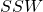and maximise, the estimator for the corresponding sample will also perform better than by using a less efficient variable. There may be practical reasons why this isn’t possible and it makes more sense to use a natural stratification variable however there are many examples where using unsupervised learning to construct a stratification variable can improve the estimator or the training set to be used for modelling. This isn’t isolated to k-means, most clustering algorithms aim to do the same thing in different ways, and each has it’s benefits given the structure of the data. This can be expanded to more sophisticated sampling techniques and not confined to simple stratified samples.

The post Improve Your Training Set with Unsupervised Learning appeared first on Daniel Oehm | Gradient Descending.